Phase of the Riemann Zeta Function and the Inverted Harmonic Oscillator (from Physical Review E, Volume 52, Number 1, July 1995, 486-491) R.K. Bhaduri, Avinash Khare, and J. Law Department of Physics and Astronomy, McMaster University, Hamilton, Ontario, Canada L8S 4M1     ABSTRACT The Argand diagram is used to display some characteristics of the characteristics of the Riemann zeta function. The zeros of the zeta function on the complex plane give rise to an infinite sequence of closed loops, all passing through the origin of the diagram. The behaviour of the phase of he zeta function on and off the line of zeros is studied. Up to some distance from the line of the complex zeros, the phase angle is shown to still retain their memory. The Argand plots also lead to an analogy with the scattering amplitude and an approximate rule for the location of the zeros. The smooth phase of the zeta function along the line of the zeros is related to the quantum density of states of an inverted oscillator.     I. INTRODUCTION The Riemann zeta function zeta(s) of the complex variable s = rho + it, defined by the equation (for rho > 0)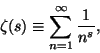has an infinite number of zeros on the half-line rho = 1/2 . These zeros are of great interest to mathematicians from the number theoretic point of view [2,3], and to physicists interested in quantum chaos and the periodic orbit theory [4-6]. Along this line, as a function of t, every time zeta(s) changes sign, a discontinuous jump by pi in the phase angle is introducted. Otherwise the phase angle is a smooth function of t. The smooth part of the phase angle itself is very interesting, since it counts the number of zeros on the1/2 line fairly accurately. In this paper we bring out some of the interesting properties of zeta(s) by displaying it on an Argand diagram, where Re(zeta(s)) (along the x axis), is plotted against Im(zeta(s)) (y axis). For rho = 1/2, the plot yields an infinite sequence of closed loops, one for every zero of the zeta function, all going through the origin. We show that as one moves somewhat away from the rho = 1/2 line, the phase angle still has sharp drops at those values of t which hve zeros on the 1/2 axis. However, this "memory" of the zeros begins to fade as one moves along the real axis. We also point out the similarity in the Argand diagrams for zeta(1/2 + it) and the resonant quantum scattering amplitude, and this analogy, although flawed, leads directly to an approximate quantization condition for the location of the zeros [7,8]. We demonstrate that for rho = 1/2, the smooth part of the phase angle of zeta(s) is closely related to the phase shift of an inverted half harmonic oscillator. II. THE PHASE ANGLE AND THE ARGAND DIAGRAM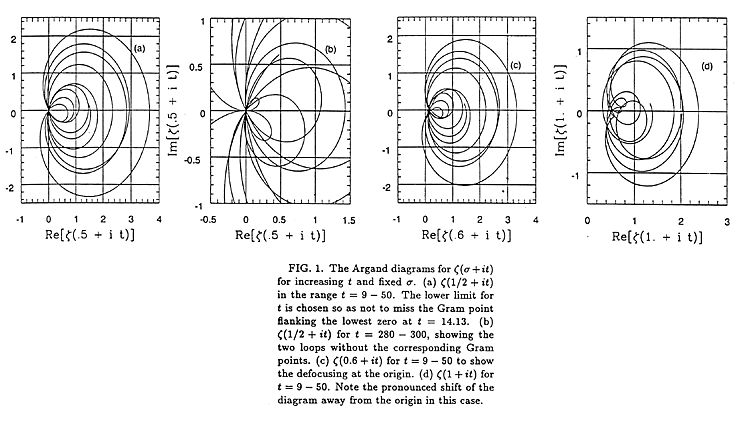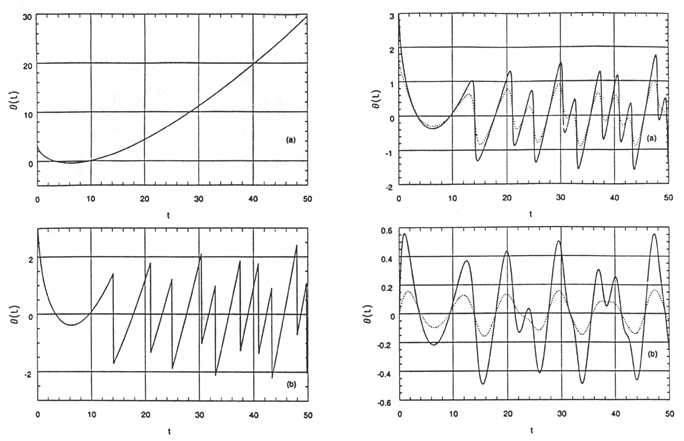IV. THE INVERTED HARMONIC OSCILLATOR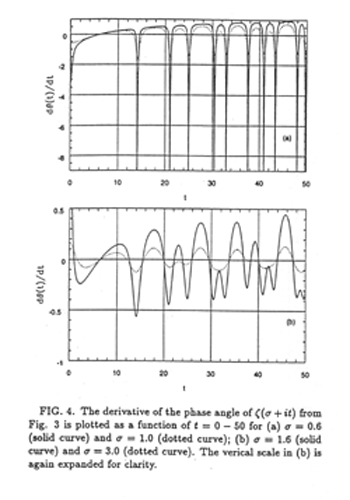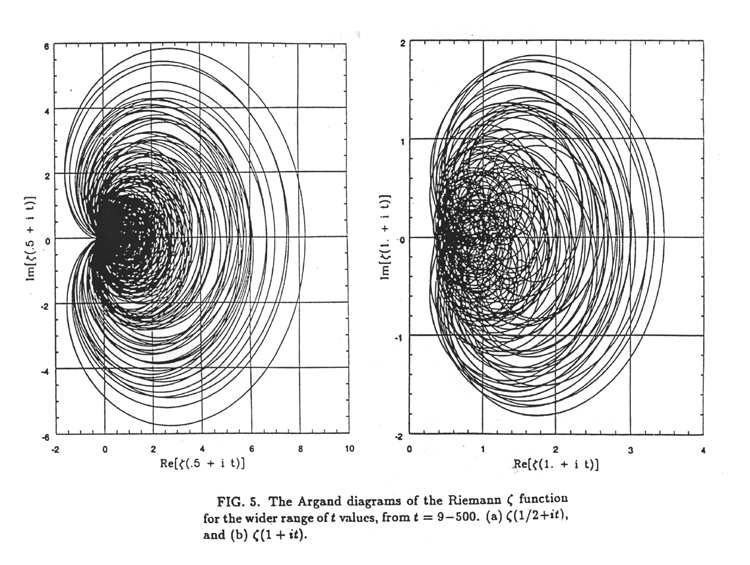V. SUMMARY In summary, we recapitulate the main points made in this paper. Traditionally, the behavior of the zeros of the Riemann zeta function on the 1/2 axis is associated with the bound state problem of a quantum Hamiltonian. We, on the other hand, focus on the scattering problem. We use the Argand diagram construction for the Riemann zeta function to visualize how the smooth phase theta(t) acts as a counter for the zeros of Z(t). Off the 1/2 axis, the zigzag pattern of the phase shift as a function of t transforms to smooth undulations which still retain the memory of the zeros. This memory fades, however, as the distance from the 1/2 axis increases. We also note from the Argand diagrams of the zeta function its analogy with the scattering amplitude, and the approxiate conditions [Eq.(9)] for the location of the zeros. The smooth part of the phase angle of the zeta function on the rho =1/2 line is related to the quantum phase shift of a potential. It is perhaps not surprising that this potential that generates the smooth phase is as simple as the inverted harmnonic oscillator. The chaotic jumps in the phase, shown in Fig. 2(b), have been left out from this. It is known that for the chaotic phase at rho = 1 [9-11], the motion of the particle is on the surface of a saddle. The orbits in this case are all unstable. It is as if the smooth phase on the1/2 line still remembered a section of the saddle, i.e., the inverted oscillator. Finally, we suggest that the Argand diagram construction may also be useful for the Selberg zeta function, and shed light on the quantization condition for quantum chaos . This last point is under current investigation.   REFERENCES  G.H. Hardy, Comptes Rendus 158 (1914), 280.  H.M. Edwards, Riemann's Zeta Function (Academic, New York, 1974).  A. Ivic, The Riemann Zeta Function (Wiley, New York, 1985).  M.V. Berry, Proceedings of the Royal Society of London, Series A400 (1985), 229.  M.C. Gutzwiller, Chaos in Classical and Quantum Mechanics, Springer-Verlag, New York, 1991.  Hua Wu and D.W.L. Sprung, Physical Review E 48 (1993), 2595.  M.V. Berry, in Quantum Chaos and Statistical Nuclear Physics, edited by T.H. Seligman and H. Nishioka, Lecture Notes in Physics, Volume 263 (Springer Verlag, New York, 1986) p.1.  R. Aurich and F. Steiner, Physical Review A 45 (1992), 583.  B.S. Pavlov and L.D. Faddeev, Sov. Math.3 (1975), 522.  P. Lax and R.S. Philips, Scattering Theory for Automorphic Functions (Princeton University Press, Princeton, N.J., 1976)  M.C. Gutzwiller, Physica D 7 (1983), 341.   archive      tutorial      mystery      new      search      home      contact